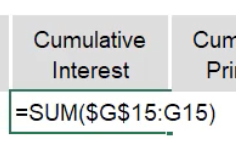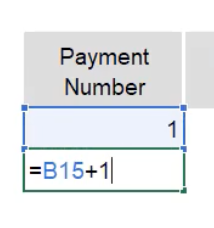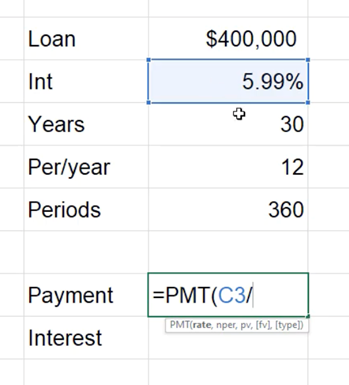# Loan Amortization Schedule

In this chapter, you will build a loan amortization schedule. You’re building this to gain a better understanding of what’s called the time value of money. The basic principle of the time value of money is that a dollar today is worth more than a dollar tomorrow, and that interest can work for or against you.

Use this workbook for the chapter.

## Entering Inputs

There is a chance you may need to take out a loan at some point, so we will do a simple example of an amortization schedule for a car loan. In this case, you will be buying a 1990 Honda Accord in North America. Fill out the input information as found in Figure 34.1.

Let’s start by understanding what the Scheduled payment for this type of loan would be. There’s a formula called PMT. The PMT function calculates the payment for a loan based on constant payments and a constant interest rate.

1. Select the box next to Scheduled payment.
2. Type =PMT(.
1. The first number it will ask you to enter is the rate.
2. If you open up the dialogue box on the ribbon, it says, “Rate is the interest rate per period for the loan. For example, use 6%/4 for quarterly payments at 6% APR.”
3. For the rate, take the annual interest rate divided by twelve payments per year.
4. Then, it wants to understand the number of periods (nper). There are two years multiplied by twelve payments per year.
5. The present value (pv)  of the loan is the loan amount.
6. The future value (fv) will eventually be zero. Leave the type option argument blank.

This formula results in a scheduled payment of $68.49 in order to start paying off this amount. The Scheduled number of payments box provides an opportunity to understand how many payments it will actually end up being. 1. In the box for Scheduled number of payments, multiply the loan period by the payments per year, which will be 24 payments. The Actual number of payments might vary based on the table below. The number of early payments will also be determined based on the table below. The last box would calculate the total interest that we would pay if we don’t pay anything extra or early. To calculate the Total interest, you will use the Cumulative Interest formula (=CUMIPMT). 1. The rate again is the interest rate divided by the number of payments in a year. 2. The number of periods would be the loan period in years multiplied by the payments per year. 3. The present value of the loan is the loan amount. 4. For the start period and end period, if you select the function arguments button, it will tell you that the start period is the first period of the calculation. In this case, enter 1 for the start period. It also says the end period is the last period. We know that there are 24 scheduled payments, so enter 24 for the end period, and select OK 5. The type asks when you’re going to pay. Select beginning of the period, and push enter 6. Use the format painter on the total interest, and you will find that you’re going to pay$45.72 in interest over the life of this loan.

## Calculate the First Row

Now, navigate to enter in the first row of the schedule.

1. So, in the first row of the schedule, we’ll calculate the payment number as payment number one, so type 1
2. The payment date will be equal to the start date.
3. The beginning balance will be equal to the loan amount. The scheduled payment will always be the same, so link this cell to the scheduled payment cell above.
4. In order to calculate the principal, we need to first know the interest.
1. To get to the interest, take the beginning balance multiplied by the annual interest rate.
2. Anchor the annual interest rate, then divide by the number of payments in a year, which will also need to be anchored, and then press Enter
5. The principal is equal to the difference between our scheduled payment minus the interest. Since scheduled payment is entered as a negative number per this formula, place a negative sign in front of the PMT in the initial scheduled payment.
1. The reason it’s negative is because it denotes a loan, and you’re paying this money out.
2. Make sure that that is switched correctly in the summary calculations as well as the schedule.
3. So, of the $68 that we pay,$64 goes to principal and $3.95 goes to interest. 6. In the first period, let’s say that we’re not going to make any extra payments, so for that, enter 0. 7. The total payment will be equal to the sum of that extra and our scheduled payment. 8. The ending balance of the loan will be equal to the beginning balance minus the principal portion and the extra payment. 9. At this point, our cumulative interest is the amount shown in Interest. Use the sum function found in Figure 34.2, and be sure to lock only the very first argument. 1. That enables the capacity to add cumulatively as you go when it is dragged down. 10. Do the same thing for the cumulative principal using the sum function. 1. Start with the principal amount, press the colon ( : ) key, and close parentheses. 2. In the formula bar, be sure to and edit it in order to lock the first argument.Figure 34.2 ## Calculate the Second Row 1. For payment number two, select the first cell and add one. (See Figure 34.3) 2. For the payment date, use the EDATE formula, and choose to add a single month. 1. You will have to update the formatting. Use CTRL+Shift+3, to put it into a date format. 3. The beginning balance is equal to the ending balance from the previous period. 4. The scheduled payment will always remain the same. Use the L6 that you’ve referenced previously and if you anchor that, you can drag that formula down. Copy and paste the formula only. 1. Again, you may need to use the format painter to format it as currency. 5. The principal is going to follow the same calculation from before. 6. The interest will also follow that same calculation since the cells at the top have been locked. 7. Extra payment again will be zero. 8. Total payment will be the same formula. 9. Ending balance will be the same formula. 10. Cumulative interest and cumulative principal will also be the same. 1. You can see in the formula that both what you paid last period and what you’re paying this period is being added.Figure 34.3 ## Calculating the Rest Now that the second row is built, you can copy the row down the number of periods that you have. In this case, there are 24 periods, so you will need 24 rows. You’ll notice that the beginning balance actually goes negative. The reason for that is we started paying extra here because the formula started adding for us. If you delete all the values from the beginning balance column, you’ll see that you end up paying off the loan and have an ending balance exactly at the end of period 24, which is what we had set in our loan calculation. These are the basics of how to make a loan amortization schedule. ## Extra Payments Let’s say you paid$100 extra in the first period. By doing so, you’ll notice that it actually goes negative in the 24th period. You finished paying off the loan in the 23rd period. And so, when you navigate to the actual number of payments, you can use the =COUNTIFS formula.

1. Type =COUNTIFS, and select the criteria range of the beginning balance column.
2. Next, enter that this range is greater than zero.

The number of early payments, also sometimes called extra payments, would use the =COUNT formula of the extra payments column. Because there only is one extra payment, you’ll see that cut off an extra period. If you were to pay another extra $100 in period two, you’ll notice that it cut off three total periods. At the end of this, at the bottom of the page, you’ll be able to see that, in fact, you finished paying it off in period 21 ## Interest Loans can be fairly straightforward in terms of how these calculations work. The important function that you need to know is the payment function (=PMT), where you can calculate how much you’ll owe given very simple inputs. You also need to understand the total amount of interest that you end up paying over the life of a loan. In fact, if you look at what was done by paying$100 extra for those first two periods, you can see that the cumulative interest paid was $38.74 since you would not calculate anything after you’ve paid off the loan. If you calculate the difference between the total interest that you were supposed to pay over the life of the loan compared to the interest that you actually ended up paying down, it’s actually a 15% savings in interest. Now, it may not seem like that much when the dollars are relatively small like this, but over the course of a large loan, like a home loan, where the dollar amounts would potentially be in the hundreds of thousands, the amount of interest that you could save would be in the tens of thousands. So, knowing how to make these calculations work and how to create one of these schedules is a vital skill to have for anyone that will borrow money at any point in their lives. That could be on a credit card, student loans, home loans, car loans, or any type of money borrowing. You should understand how financial institutions charge you money to use their funds. ## Potential Home Loan Valuation Let’s learn a little bit about potential home loan valuation. Given just a very little bit of information, you can use the payment calculation to calculate what you might owe for a given home that you’re considering purchasing. (See Figure 34.4)Figure 34.4 To find the payment, using the payment function, take the interest rate divided by the number of periods per year, the number of periods total, the present value of the loan, and the future value, which will be 0. You’ll see that the payment for this loan would be$2,395.63 per month, just to cover the loan.

You can also quickly calculate the amount of interest that you’ll pay over the life of this loan. Using the =CUMIPMT function, divide the rate by the number of periods per year, select the number of total periods and the present value of the loan, enter start period 1, ending period of 360, and select that you’ll pay at the beginning of the period. Over the course of this loan, you will pay more than the value of it originally if you keep the interest rate of 5.99% and keep your paying minimum amount of $2,395.63. Just to show you how valuable this might be, make a copy of these numbers and change the interest rate from 5.99% to 4.99%. The difference in what you pay in interest is$90,000 savings. If you copy it again and bring it down to 3.99%, you save an additional $85,000 against the previous 4.99% interest rate. If you copy it one last time and change the interest to 2.99%, this saves us an additional$80,000 against the 3.99% interest. Against the original 5.99%, you’ve saved \$256,000.

However, in this example, that still means that you’ve paid that amount for your home plus the interest that you paid over the life of the loan. So, you’ve paid for this home more than it’s worth. If we divide what you paid by the value of the home, you’ve paid for it 152%. You have quite the ownership in your property at that point.

Hopefully, this example serves to illustrate how important the time value of money is and how much you can end up paying or saving, because of course, these numbers could be applied to a savings account that you’re earning interest on over time and the opposite effect would be the case.